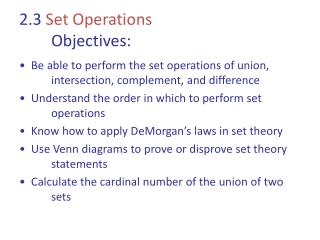DownloadDownload Presentation2.3 Set Operations Objectives:

# 2.3 Set Operations Objectives:

Download Presentation## 2.3 Set Operations Objectives:

- - - - - - - - - - - - - - - - - - - - - - - - - - - E N D - - - - - - - - - - - - - - - - - - - - - - - - - - -
##### Presentation Transcript

1. 2.3 Set Operations Objectives: • Be able to perform the set operations of union, intersection, complement, and difference • Understand the order in which to perform set operations • Know how to apply DeMorgan’s laws in set theory • Use Venn diagrams to prove or disprove set theory statements • Calculate the cardinal number of the union of two sets

2. Union of Sets

3. Union of Sets cont. • Example: Find the union of the two sets. • Find the union of the two sets. • A = { x : x is the opposite of a natural number } • B = { x : x is a whole number } ____________________________________________ ?

4. Intersection of Sets

5. Intersection of Sets cont. • Example: Find the intersection of the two sets. • Find the intersection of the two sets. • A = { x : x is the opposite of a natural number } • B = { x : x is a whole number } ____________________________________________ ?

6. Set Complement Note: Think of A complement as not A .

7. Set Complement cont. • Example: Given U and A, find the complement of A. • Given U and A, find the complement of A. • U = { x : x is a real number } • A = { x : x > 3.1 } • A’ = _________________________________ ?

8. Set Difference B - A Note 1: Think of B – A as what is in B “take away” what is in A . Note 2: B – A A – B !

9. Set Difference cont. • Example: Find the difference. • Find the differences. • A = { x : x is an integer } • B = { x : x is a natural number } • A – B = ________________________________ ? • B – A = ________________________________ ?

10. Order of Set Operations • Similar to Order of Operations for arithmetic. • Example: Let

11. Order of Set Operations cont. • Similar to Distributive Property of Multiplication over Addition. a( b + c ) = ________________________ ? • Intersection distributes over union. • Use Venn diagrams to show

12. DeMorgan’s Laws Use Venn diagrams to prove DeMorgan’s Laws for sets.

13. Cardinality of a Union Let A = { 1, 2, 3 } and B = { 3, 4, 5 } Then _________________________ ? and n( ) = _________ ? n ( ) =